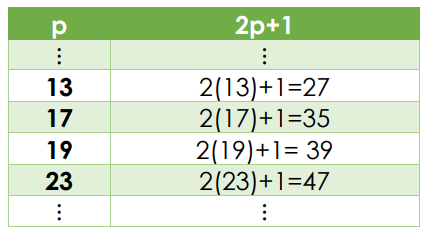# Is 43 a prime number?How many random people should be gathered to ensure that at least five of them will know each other, or at least five of them will be complete strangers to each other?

You guessed right, 43 people are needed! Another property of 43 is that it is a prime number, as we will see next.

We will start recalling some definitions that we have widely discussed in our Prime Numbers article. If you still feel unfamiliar with these notions, we invite you to first read that article and come back here later.

A factor of a natural number is a positive divisor of the number. A proper factor of a natural number is a factor that is different from 1 and from the number itself. For example, 34 = 2×17 = 1×34; thus, 1, 2, 17, and 34 are all factors of 34, but only 2 and 17 are proper factors of 34.A natural number is called a prime number if it is greater than 1, and it doesn’t have proper factors. For example, the four prime numbers below 43 are 29, 31, 37, and 41.

A composite number is a natural number that has proper factors. As we saw, 34 has two proper factors, thus 34 is a composite number.## Why is 43 a prime number?

Number 43 is prime because it doesn’t have proper factors. In other words, the only factors of 43 are 1 and itself. To be sure of it, we can use the following property.

If 𝒏 is a natural number, and neither of the prime numbers less than $$\sqrt n$$ divides 𝒏, then 𝒏 is a prime number.Notice that 43<49, thus $$\sqrt{43}\;<\;\sqrt{49}\;=\;7$$. Therefore, the prime  umbers less than $$\sqrt{43}$$ are 2, 3 and 5.

Since 43 isn’t an even number, 2 doesn’t divide 43. Moreover, 43 = (3×14) + 1 and 43 = (5×8) + 3, meaning that neither 3 nor 5 divides 43. Then, by the property above, 43 is a prime number.

On the other hand, a prime number of objects can’t be arranged into a rectangular grid with more than one column and more than one row. This is another way of verifying that 43 is a prime number:

• For example, if we try to arrange 43 stars into a rectangular grid with four rows, one of the columns will be incomplete.The same happens if we try to arrange 43 stars into a rectangular grid with any number of rows and columns greater than one.
• The only way of arranging 43 stars into a rectangular grid, is by having a single row, or a single column. This means that 43 is a prime number!

## Which class of prime number is 43?

Since 43=41+2, and 41 is also a prime number, 43 is a twin prime: this is, a prime number that is 2 less or 2 more than another prime number. Also, 43 is the smallest prime number that can be written as the sum of 2, 3, 4 or 5 different primes:

43 = 41 + 2
43 = 11 + 13 + 19
43 = 2 + 11 + 13 + 17
43 = 3 + 5 + 7 + 11 + 17

Forty-three can be classified into several classes of primes numbers. However, as we will see next, it doesn’t belong to any of the three classes that we mention below.

 Classes of Prime Numbers Primoral prime It is a prime number of the form $$\\p=\left(p_1\times p_2\times p_3\times…\times p_n\right)+ 1$$, or $$\\p=\left(p_1\times p_2\times p_3\times…\times p_n\right)- 1$$  where $$p_{1,\;\;}p_{2,\;\;}p_{3,\;…,}\;p_n\;$$  are the first n prime numbers. No Mersenne prime It is a prime number of the form $$p=2^n-1$$where n is an integer. No Safe prime It is a prime number of the form  2p+1  where p is also a prime number. No

Let’s find out why:

• Using the first three prime numbers 2, 3 and 5 in the primoral formula, we get (2 × 3 × 5) − 1 = 29 and (2 × 3 × 5) + 1 = 31. Using the first four primes 2, 3, 5, and 7, we get (2 × 3 × 5 × 7) − 1 = 209 and (2 × 3 × 5 × 7) + 1 = 211. Since the first two of the resulting numbers are less than 43, and the last two are greater than 43, then 43 doesn’t have the form of a primoral prime.
• Notice that:
$$2^5\;\;-\;1\;=\;32\;-\;1\;=\;31\;\\{2^6\;\;}-\;1\;=\;64\;-\;1\;=\;63$$
Therefore, 43 doesn’t have the form of a Mersenne prime.
• Let’s consider the values that take 2p+1, for different primes p:Since 43 is not in the right column, it doesn’t have the form of a safe prime.

We invite you to read other articles on prime numbers, on our blog, to find out which other prime numbers belong to these classes.

Do you know that 41 is also a prime number?